# How to Generate All Permutations in Excel

This tutorial demonstrates how to generate all permutations in Excel.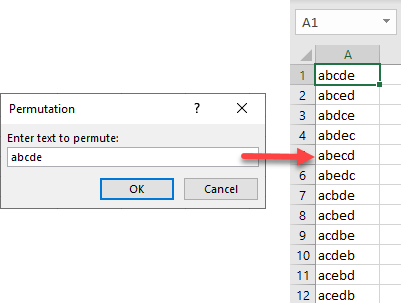## Generate All Permutations

Using a VBA macro, you can generate all possible permutations of a given set of characters. All you need to do is create a small piece of code and enter the input characters.

1. In the Ribbon, go to Developer > Visual Basic. If you don’t have this tab available, find out how to add the Developer tab.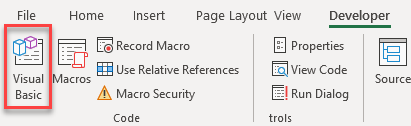1. In the VBA window, go to Insert > Module.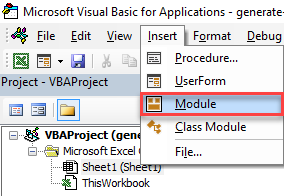1. In the code window on the right side, paste the following code and save.
``````Sub GetString()
Dim xStr As String
Dim FRow As Long
Dim xScreen As Boolean
xScreen = Application.ScreenUpdating
Application.ScreenUpdating = False
xStr = Application.InputBox("Enter text to permute:", "Permutation", , , , , , 2)
If Len(xStr) < 2 Then Exit Sub
If Len(xStr) >= 8 Then
MsgBox "Too many permutations!", vbInformation, "Permutation"
Exit Sub
Else
ActiveSheet.Columns(1).Clear
FRow = 1
Call GetPermutation("", xStr, FRow)
End If
Application.ScreenUpdating = xScreen
End Sub
Sub GetPermutation(Str1 As String, Str2 As String, ByRef xRow As Long)
Dim i As Integer, xLen As Integer
xLen = Len(Str2)
If xLen < 2 Then
Range("A" & xRow) = Str1 & Str2
xRow = xRow + 1
Else
For i = 1 To xLen
Call GetPermutation(Str1 + Mid(Str2, i, 1), Left(Str2, i - 1) + Right(Str2, xLen - i), xRow)
Next
End If
End Sub
``````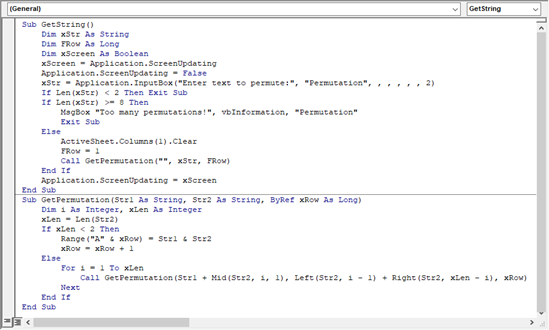1. Now you have created the GetString macro, and you can run it. In the Ribbon, go to Developer > Macros.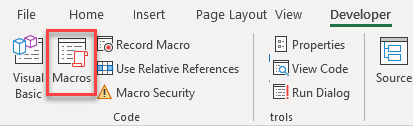1. If it’s not already selected, select the macro, and click Run.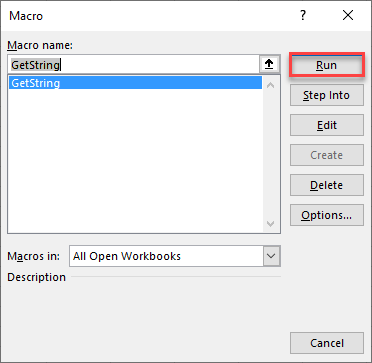1. In the pop-up window, enter characters for permutation (here, abcde), and click OK.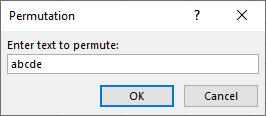As a result, in Column A, you get all possible (120) permutations of entered characters.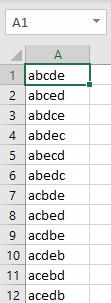In addition to the macro described above, Excel has a built in function, the PERMUT Function, which enables you to calculate how many permutations occur for any given numbers. This function only works with numbers and not with text.